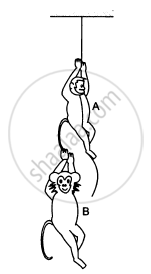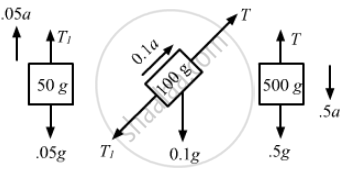Advertisement Remove all ads

# Find the Acceleration of the 500 G Block in the Following Figure . - Physics

Sum

Find the acceleration of the 500 g block in the following figure.Advertisement Remove all ads

#### Solution

Given,
m1 = 100 g = 0.1 kg
m2 = 500 g = 0.5 kg
m3 = 50 g = 0.05 kg

The free-body diagram for the system is shown below:From the free-body diagram of the 500 g block,
T + 0.5a − 0.5g = 0                    .....(i)

From the free-body diagram of  the 50 g block,
T1 + 0.05g − 0.05a = a               ....(ii)

From the free-body diagram of the 100 g block,
T1 + 0.1a − T + 0.5g = 0           ....(iii)

From equation (ii),
T1 = 0.05g + 0.05a                 .....(iv)

From equation (i),
T1 = 0.5g − 0.5a                     .....(v)

Equation (iii) becomes
T1 + 0.1a − T + 0.05g = 0

From equations (iv) and (v), we get:
0.05g + 0.05a + 0.1a − 0.5g + 0.5a + 0.05g = 0
0.65a = 0.4 g

$\Rightarrow a = \frac{0 . 4}{0 . 65}g$

$= \frac{40}{65}g = \frac{8}{13}g \left(\text{ downward }\right)$

So, the acceleration of the 500 gm block is
$\frac{8g}{13}$ downward.

Is there an error in this question or solution?
Advertisement Remove all ads

#### APPEARS IN

HC Verma Class 11, 12 Concepts of Physics 1
Chapter 5 Newton's Laws of Motion
Q 35 | Page 82
Advertisement Remove all ads
Advertisement Remove all ads
Share
Notifications

View all notifications

Forgot password?